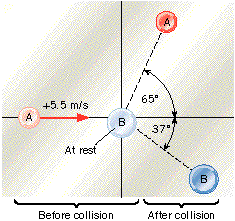# Problem: The drawing shows a collision between two pucks on an air-hockey table. Puck A has a mass of 3.5 kg and is moving along the x axis with a velocity of +5.5 m/s. It makes a collision with puck B, which has a mass of 6 kg and is initially at rest. After the collision, the two pucks fly apart with the angles shown in the drawing.(a) Find the final speed of:puck A:  m/spuck B:  m/s(b) Find the kinetic energy of the A+B system:before the collision:  Jafter the collision:  JWhat type of collision is this?

###### FREE Expert Solution

In this problem, we're going to use the law of conservation of linear momentum.

Momentum:

$\overline{){\mathbf{p}}{\mathbf{=}}{\mathbf{m}}{\mathbf{v}}}$, where p is momentum, m is mass, and v is velocity.

We now write the law of conservation of linear momentum in the x-direction as:

$\overline{)\begin{array}{rcl}{\mathbf{m}}_{\mathbf{A}}{\mathbf{v}}_{\mathbf{0}\mathbf{A}}& {\mathbf{=}}& {\mathbf{m}}_{\mathbf{A}}{\mathbf{v}}_{\mathbf{f}\mathbf{A}}\mathbf{c}\mathbf{o}\mathbf{s}\mathbf{\left(}\mathbf{65}\mathbf{°}\mathbf{\right)}\mathbf{+}{\mathbf{m}}_{\mathbf{B}}{\mathbf{v}}_{\mathbf{f}\mathbf{B}}\mathbf{c}\mathbf{o}\mathbf{s}\mathbf{\left(}\mathbf{37}\mathbf{°}\mathbf{\right)}\end{array}}$

In the y-direction, we have:

$\overline{){\mathbf{0}}{\mathbf{=}}{{\mathbf{m}}}_{{\mathbf{A}}}{{\mathbf{v}}}_{\mathbf{f}\mathbf{A}}{\mathbf{s}}{\mathbf{i}}{\mathbf{n}}{\mathbf{\left(}}{\mathbf{65}}{\mathbf{°}}{\mathbf{\right)}}{\mathbf{-}}{{\mathbf{m}}}_{{\mathbf{B}}}{{\mathbf{v}}}_{\mathbf{f}\mathbf{B}}{\mathbf{s}}{\mathbf{i}}{\mathbf{n}}{\mathbf{\left(}}{\mathbf{37}}{\mathbf{°}}{\mathbf{\right)}}}$

Kinetic energy:

$\overline{){\mathbf{K}}{\mathbf{=}}\frac{\mathbf{1}}{\mathbf{2}}{{\mathbf{mv}}}^{{\mathbf{2}}}}$

We can solve for vfB from the equation of conservation of linear momentum in the y-direction equation as:

$\begin{array}{rcl}{\mathbf{m}}_{\mathbf{B}}{\mathbf{v}}_{\mathbf{fB}}\mathbf{sin}\mathbf{\left(}\mathbf{37}\mathbf{°}\mathbf{\right)}& \mathbf{=}& {\mathbf{m}}_{\mathbf{A}}{\mathbf{v}}_{\mathbf{fA}}\mathbf{sin}\mathbf{\left(}\mathbf{65}\mathbf{°}\mathbf{\right)}\\ {\mathbf{v}}_{\mathbf{fB}}& \mathbf{=}& \frac{{\mathbf{m}}_{\mathbf{A}}{\mathbf{v}}_{\mathbf{fA}}\mathbf{sin}\mathbf{\left(}\mathbf{65}\mathbf{°}\mathbf{\right)}}{{\mathbf{m}}_{\mathbf{B}}\mathbf{sin}\mathbf{\left(}\mathbf{37}\mathbf{°}\mathbf{\right)}}\end{array}$

87% (155 ratings)###### Problem DetailsThe drawing shows a collision between two pucks on an air-hockey table. Puck A has a mass of 3.5 kg and is moving along the x axis with a velocity of +5.5 m/s. It makes a collision with puck B, which has a mass of 6 kg and is initially at rest. After the collision, the two pucks fly apart with the angles shown in the drawing.(a) Find the final speed of:
puck A:  m/s
puck B:  m/s

(b) Find the kinetic energy of the A+B system:
before the collision:  J
after the collision:  J
What type of collision is this?